Home | ARTS | Operations Management | Construction Of Project Network Diagrams - Project Network

# Construction Of Project Network Diagrams - Project Network

Posted On :  23.06.2018 09:43 am

Construct the network diagram for a project with the following activities:

Construction Of Project Network Diagrams

Problem 1

Construct the network diagram for a project with the following activities: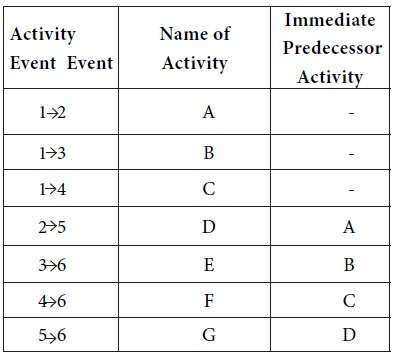Solution

The start event is node 1.

The activities A, B, C start from node 1 and none of them has a predecessor activity. A joins nodes1 and 2; B joins nodes 1 and 3; C joins nodes 1 and 4. So we get the following: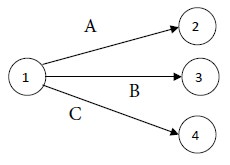This is a part of the network diagram that is being constructed. Next, activity D has A as the predecessor activity. D joins nodes 2 and 5. So we get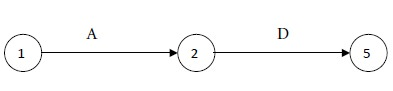Next, activity E has B as the predecessor activity. E joins nodes 3 and 6. So we get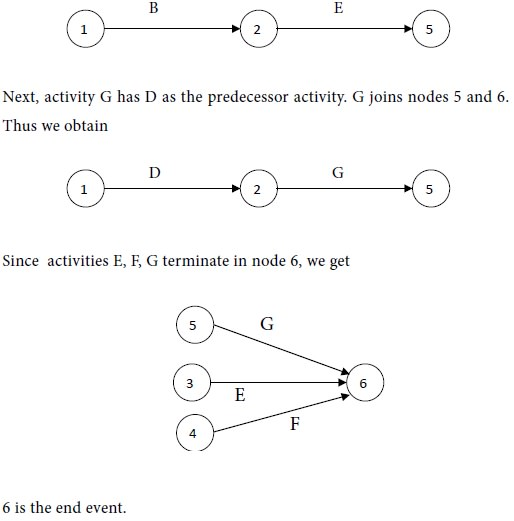Problem 2

Develop a network diagram for the project specified below:Solution

Activity A has no predecessor activity. i.e., It is the first activity. Let us suppose that activity A takes the project from event 1 to event 2. Then we have the following representation for A: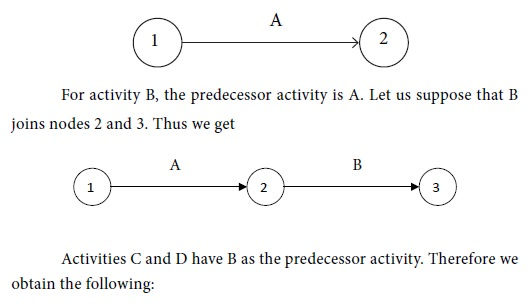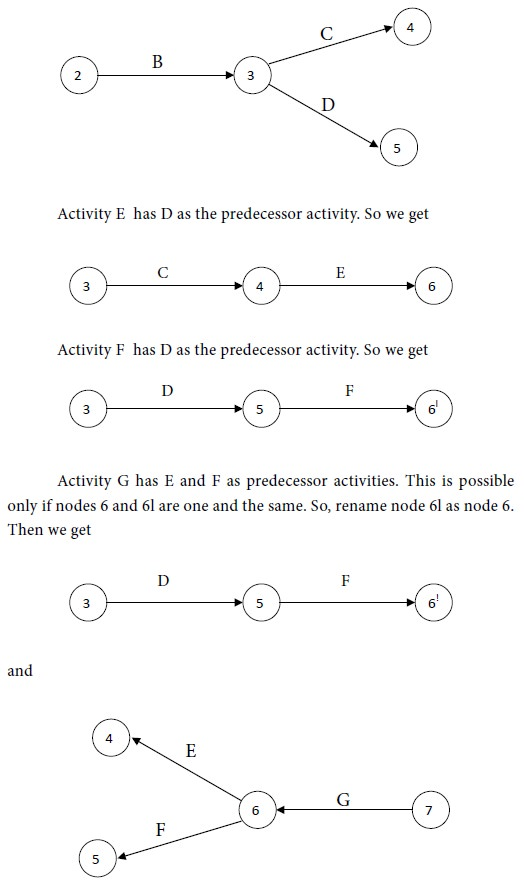G is the last activity.

Putting all the pieces together, we obtain the following diagram the project network:The diagram is validated by referring to the given data.

Note: An important point may be observed for the above diagram. Consider the following parts in the diagram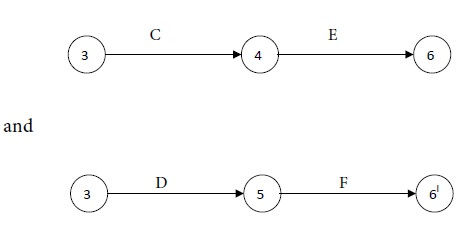We took nodes 6 and 6l as one and the same. Instead, we can retain them as different nodes. Then, in order to provide connectivity to the network, we join nodes 6l and 6 by a dummy activity. Then we arrive at the following diagram for the project network:Tags : Operations Management - Network Problems
Last 30 days 351 views